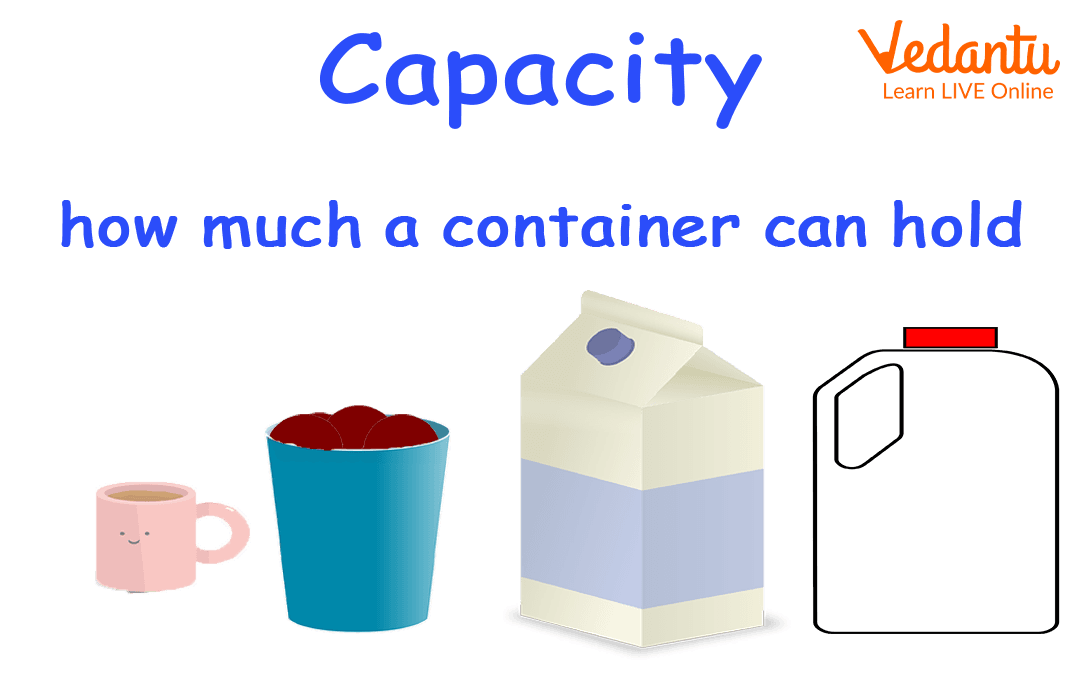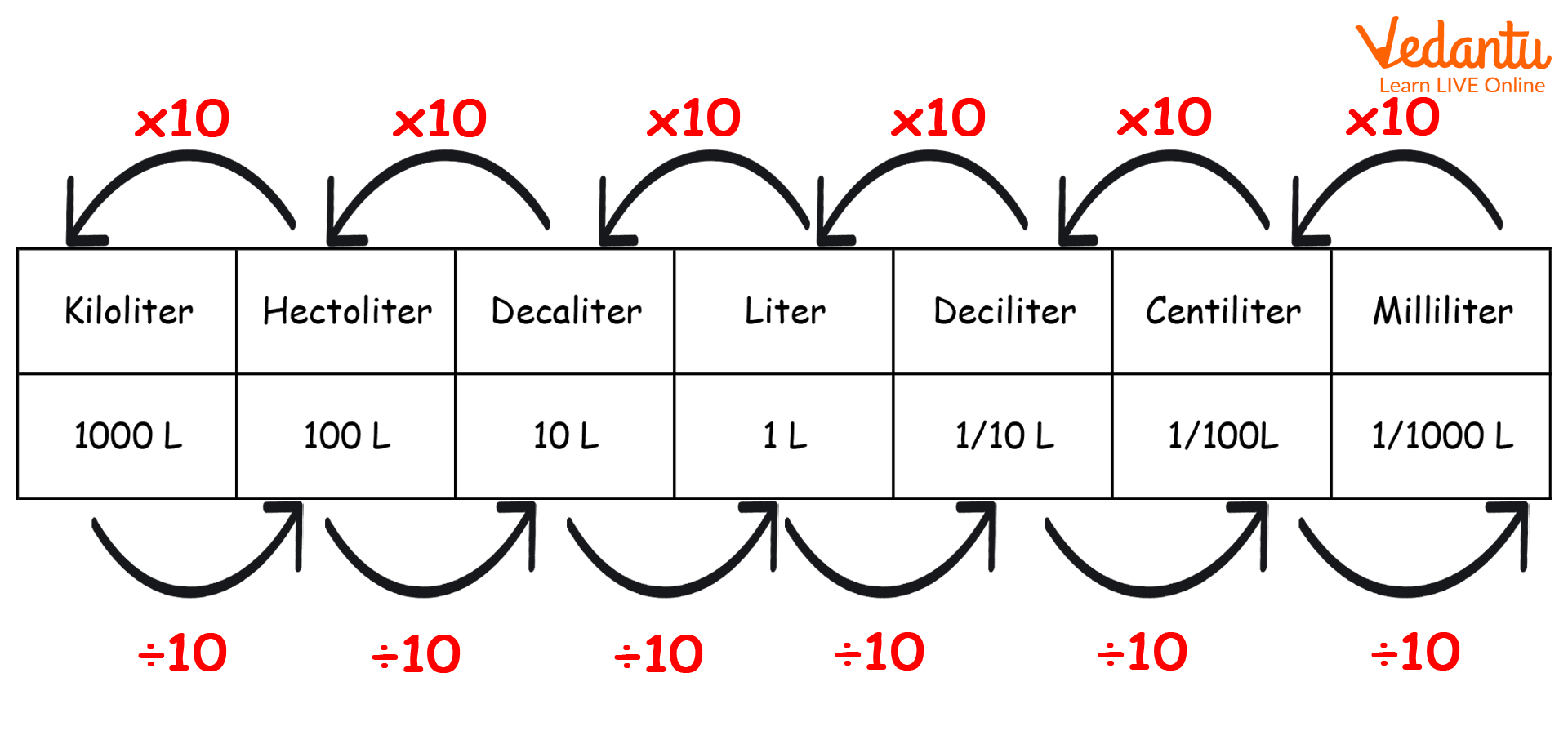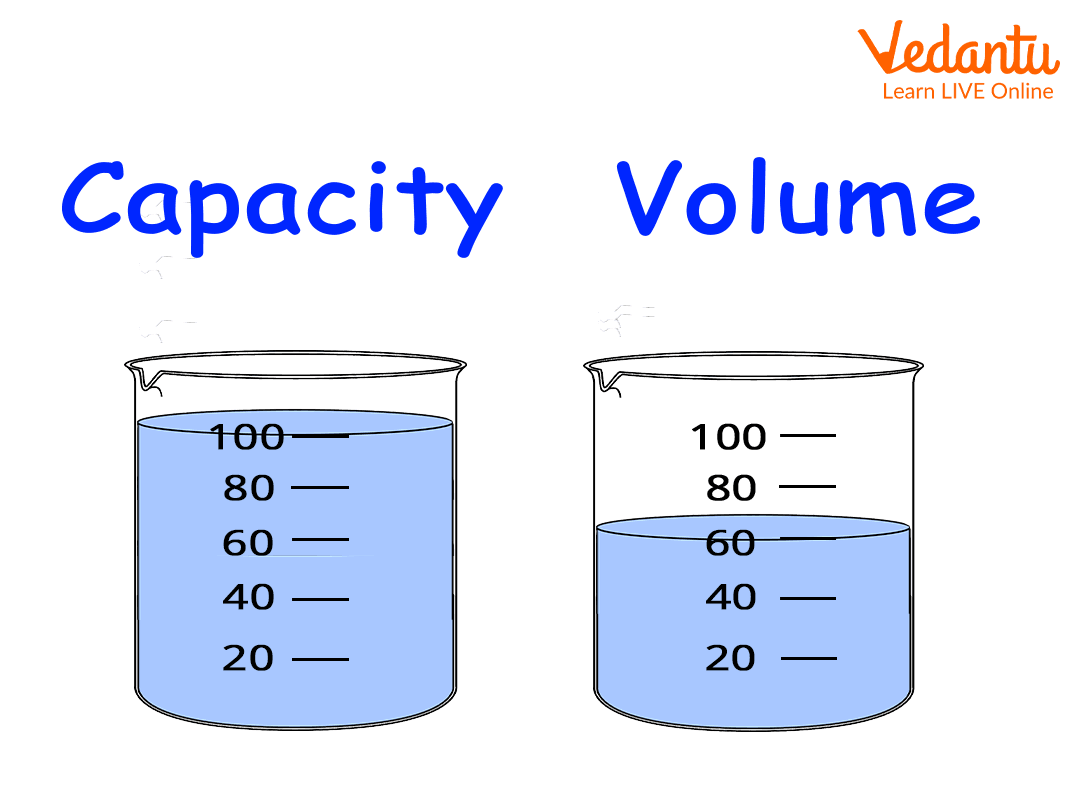Courses
Courses for Kids
Free study material
Free LIVE classes
More

# Unit of CapacityLIVE
Join Vedantu’s FREE Mastercalss

## Introduction

Capacity is an important quantity we use to measure liquids. We use different units of capacity daily. Capacity is the amount that can be held in a hollow container. We measure chemicals in millilitres and petrol in litres.

### What is Capacity?

Before we learn the units of capacity, let’s first learn what capacity is. Capacity is the amount that can be held in a hollow container. Its unit is a litre. In other words, it refers to the volume of a hollow container. We should keep in mind that volume and capacity are not the same. Volume is measured in cm3, and m3 but capacity is measured in litres or gallons.Capacity

### Unit of Capacity

The SI unit of capacity is litres. But in some places in the world, it is measured in gallons. The short term for litre is L and that for millilitre is ml. Mathematically, 1 litre $=$ 1000 millilitres. Below is given the metric chart to convert the units of capacity.Metric chart

### Difference between Capacity of a Container and Volume of Liquid in it

Volume of a liquid in a container refers to the total amount of space covered by an object in a three-dimensional space. Whereas, capacity is the amount of matter that can be held by a hollow object. For example, volume of a liquid in a container is the amount of liquid present in a container, while the capacity is the maximum amount of liquid that can be held by the same container. All three-dimensional shapes have volume whether they are solid or hollow, whereas only hollow three-dimensional shapes can have capacity otherwise they would not be able to hold any liquid or matter within themselves. To find the capacity of a hollow shape we need its volume, we pour a liquid into the hollow shape till it's full and then we can conclude that the capacity of the container is equal to the volume of liquid in it.Capacity of a container versus Volume of liquid in a container

### Converting Litre into ${\rm{c}}{{\rm{m}}^3}$

It is very important to know how to convert units of volume of liquid between litres and ${\rm{c}}{{\rm{m}}^3}$. We know that 1 litre is equal to 1000 ${\rm{c}}{{\rm{m}}^3}$. So if the volume of water in a pot is 5 litres then it has a volume of 5000${\rm{c}}{{\rm{m}}^3}$.

Great, We have learned about capacity and volume of an object. We have successfully understood the meaning of capacity and the difference between volume and capacity of shapes and containers. We learned how to convert the units of capacity into the metric system. And we know how to convert Litre into ${\rm{c}}{{\rm{m}}^3}$ and vice versa.

### Conclusion

Capacity is commonly used to measure the amount of liquid in a container. It is important to know how much 1 litre is and its other units. We also learned the conversion of different units of capacity. And also understood that volume and capacity are not the same.

### Solved Examples

1. What is the capacity of a hollow cuboid of dimensions 10 cm x 20 cm x 30 cm?

Ans. The volume of the cuboid is 6,000 ${\rm{c}}{{\rm{m}}^3}$.

We know that 1L $=$ 1,000${\rm{c}}{{\rm{m}}^3}$. So the volume of the cuboid is 6 x 1L. Hence 6,000 cm3$=$ 6L.

2. Find the volume and capacity (in metric units) of a cube of side length 20 cm.

Ans. The volume of a cube is [side]$\times$[side]$\times$[side]

Volume $= 20 \times 20 \times 20$${\rm{c}}{{\rm{m}}^3}$

Volume $= 8000$${\rm{c}}{{\rm{m}}^3}$

Now for capacity, we need to convert the ${\rm{c}}{{\rm{m}}^3}$

into a litre

We know that 1 L $=$ 1000${\rm{c}}{{\rm{m}}^3}$

Hence, capacity $=$ 8 litres.

Last updated date: 15th Sep 2023
Total views: 79.2k
Views today: 0.79k

## FAQs on Unit of Capacity

1. Why do we need capacity?

Capacity in general is the tendency of an object to hold anything. It can either be energy or physical quantity.  For example, the capacity to work is called energy. Similarly the capacity of any object to hold anything is called the maximum volume. This opens up a vast door in fields like physics and mathematics

2. What is the physical significance of capacity?

Capacity simply means the ability of someone or something to hold something. for example how many mangoes you can hold in one hand, or how many cats can fit in a box.

3. Can a solid object have capacity?

No, solid objects can not have capacity. Capacity is the amount of matter or liquid that a hollow object can hold.

4. Which is the greater capacity of a jug or the volume of water in a jug?

The volume refers to the amount of water currently present in the jug. It can be anything. While the capacity refers to the maximum amount of water it can hold in it.

5. What is an example of capacity?

An example of capacity is how many people can fit inside a room, or how many apples can fit inside a plastic bag.